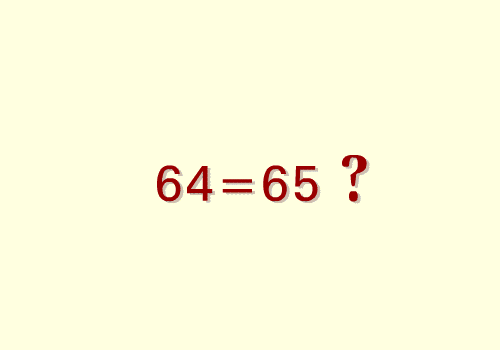Here’s a fun paradox which has been around for quite a while and was apparently a favorite of Lewis Carroll.As you can verify for yourself, the two figures above are composed of two different rearrangements of the same four pieces. However, if we compute the areas of the two figures we get a surprise: the area of the square is$8 \times 8 = 64$, whereas the area of the rectangle is$5 \times 13 = 65$! Where did the extra unit of area come from?

Feel free to post questions, comments, thoughts, guesses, etc. below. (However, please refrain from commenting if you have seen this paradox and its explanation before!)Associate Professor of Computer Science at Hendrix College. Functional programmer, mathematician, teacher, pianist, follower of Jesus.
This entry was posted in challenges, geometry, paradox, pictures and tagged , , . Bookmark the permalink.

### 18 Responses to An area paradox

1.Lynette says:

…well, the hypoteneses (hypoteni?) have different slopes, right? The yellow/green is 2/5, and the red/blue is 3/8. So the second figure doesn’t actually make squares along the red/green and yellow/blue meeting points, which is obscured by the thick black lines?

2.Elissa says:

In the 5×13, the blue square in the second row, third from the right is counted as a whole square. In the 8×8, though, it is paired with a tiny red corner that is used to complete a green square in the 5×13.

•Brent says:

You are on the right track, but that doesn’t account for a whole unit of area.

3.CalcDave says:

I have seen this before, so I will refrain from that, but I will include a link to an animated .gif where you can see the rearrangement happen if that is more/less convincing:4.Blaine says:

Just checking quickly, the slope on the green/yellow quadrilaterals is 2/5 or 0.40 while the slope on the red/blue triangles is 3/8 or 0.375. So, although the diagram shows the shapes matching nicely, I suspect the fact that the slopes are different means that there is actually a gap along the diagonal of the 5 x 13 rectangle and that gap must add up to one full square.

Now I’m off to actually compute that gap to confirm that thought…

•Blaine says:

I’m not sure if you can take links, but here is an image I created showing the gap. At its widest point, the gap is 1/8 of a unit.

You can think of the gap in 3 parts. Between the blue and yellow there is a triangle with a base of 1/8 and a height of 5 units. In the middle between the red and blue there is a parallelogram with a base of 1/8 and a height of 3 units. Finally, there is another triangle between green and red also with a base of 1/8 and height of 5 units.

First triangle = ½ bh = ½ * 5 * 1/8 = 5/16 sq. units
Parallelogram = bh = 3 * 1/8 = 3/8 sq. units
Second triangle = ½ bh = ½ * 5 * 1/8 =5/16 sq. units

Total area of the gap:
= 5/16 + 3/8 + 5/16
= 5/16 + 6/16 + 5/16
= 16/16
= 1 sq. unit

Voila!

5.xander says:

The pieces from the first figure don’t fit together in the way shown in the second figure. The non-parallel sides of the trapezoids meet at an angle of atan(2/5) (about 21.8 degrees), while the smaller angle of the triangles is atan(3/8) (about 21.6 degrees). If the red triangle and the yellow trapezoid (for instance) are put together, they would not create a right triangle similar to the red triangle as shown.

By using thicker lines for the boundaries of the shapes, you hide the fact that the diagonal of the large rectangle in the second figure does not actually pass through grid point where the red, yellow, and blue shapes meet.

•xander says:

Addendum: If we scale the red triangle up so that the short side is 5 units long, rather than 3 units, then compare it to the trapezoid, we get something that looks like this image. If the shapes from the first image are used to construct the second image, we should get something more like this. The white bit in the middle accounts for the extra square.

6.JM says:

I don’t think it’s a coincidence that all the side lengths are Fibonacci numbers. From what I can tell…

Fn^2=F(n-1)*F(n+1)+/-1

In other words, any Fibonacci number squared is one away from the product of the two adjacent Fibonacci numbers, with the plus/minus alternating each time. The fact that the slopes all approach a constant helps out as well.

7.Ryan says:

The angles are slightly different. The acute angle in the yellow/green piece is tan-1(13/5) in the 5×13, where it’s tan-1(5/2) in the 8×8. Obviously the triangles have different angles, too. So they aren’t the same pieces at all.

8.Guillermo Bautista says:

This puzzle has given me a headache when I was still in grade school.

9.fantasticsid says:

The “square blocks” in the second figure, they are not perfect squares, they are actually rectangles with very close width and length. I think this might be the cause of the illusion?

10.jd2718 says:

I’ve seen this before (got tricked by a similar problem in 9th grade geometry – didn’t solve that one).

I think I would call it a trick rather than a paradox. The trick is showing that there is no paradox.

Jonathan

•Brent says:

•jd2718 says: